# Three Ways to Get the Last Row Number in a Range

This post explains that how to get the last row number in a range or a table in excel. Sometimes, you want to know the last row number for a excel range. The below will guide you how to get the last row number in range using excel formula through the following three ways:

## Get the Last Row Number using ROW and ROWS Functions

If you want to get the last row number in a range, you need to know the first row number and the total rows number of a range, then perform the addition operation, then subtract 1, the last result is the last row number for that range.

We have talked that how to get the first row number in the previous post. And how to get the total row numbers in a range? And you can use the ROWS function. So we can write down the following formula to get the last row number in a range (assuming that B3:D6):

`=ROW(B3:D6) + ROWS(B3:D6)-1`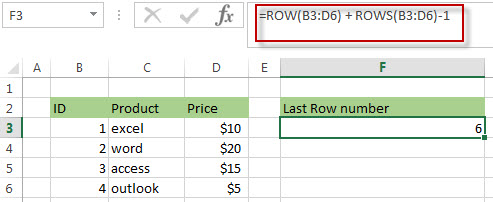The above ROW function returns the first row number in range B3:D6.

=ROWS(B3:D6)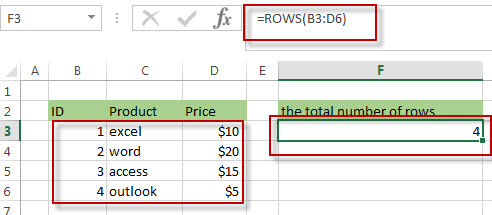The ROWS function returns the total number of rows in range B3:D6.

## Get the Last Row Number using MIN, ROW and ROWS Functions

To get the first row number, you can also use the ROW function within MIN Function as follows:

`=MIN(ROW(B3:D6))`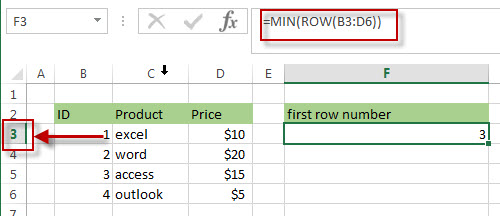So we can use the above formula to create a new formula to get the last row number in a range as follows:

`=MIN(ROW(B3:D6)) + ROWS(B3:D6)-1`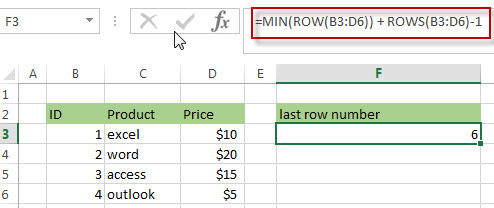## Get the Last Row Number using ROW, INDEX and ROWS Functions

As I said in the previous post, we can use the INDEX function in combination with ROW function to get the first row number in a range. So we can write down another different formula to find the last row number in a range, such as: B3:D6.

`=ROW(INDEX(B3:D6,1,1)) + ROWS(B3:D6)-1`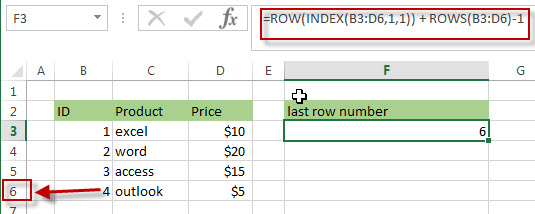### Related Formulas

• Split Text String to an Array
If you want to convert a text string into an array that split each character in text as an element, you can use an excel formula to achieve this result. the below will guide you how to use a combination of the MID function, the ROW function, the INDIRECT function and the LEN function to split a string…
• Find the Relative Position in a Range or Table
If you want to know the relative row position for all rows in an Excel Range (B3:D6), you can use a excel Array formula as follows:=ROW(B3:D6)- ROW(B3) + 1. You can also use another excel array formula to get the same result as follows:=ROW(B3:D6)-ROW(INDEX(B3:D6,1,1))+1…
• Get the First Row Number in a Range
If the ROW function use a Range as its argument, it only returns the first row number.You can also use the ROW function within the MIN function to get the first row number in a range. You can also use the INDEX function to get the reference of the first row in a range, then combined to the ROW function to get the first row number of a range.…

### Related Functions

• Excel INDEX function
The Excel INDEX function returns a value from a table based on the index (row number and column number)The INDEX function is a build-in function in Microsoft Excel and it is categorized as a Lookup and Reference Function.The syntax of the INDEX function is as below:= INDEX (array, row_num,[column_num])…
• Excel ROW function
The Excel ROW function returns the row number of a cell reference.The ROW function is a build-in function in Microsoft Excel and it is categorized as a Lookup and Reference Function.The syntax of the ROW function is as below:= ROW ([reference])….
• Excel ROWS function
The Excel ROWS function returns the number of rows in a cell reference.The ROWS function is a build-in function in Microsoft Excel and it is categorized as a Lookup and Reference Function.The syntax of the ROWS function is as below:=ROWS(array)….
• Excel MIN function
The Excel MIN function returns the smallest numeric value from the numbers that you provided. Or returns the smallest value in the array.The MIN function is a build-in function in Microsoft Excel and it is categorized as a Statistical Function.The syntax of the MIN function is as below:= MIN(num1,[num2,…numn])….